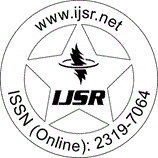International Journal of Science and Research (IJSR)
Call for Papers | Fully Refereed | Open Access | Double Blind Peer Reviewed

Research Paper | Mathematics | India | Volume 7 Issue 4, April 2018

# Eccentricity based Geometrical-Arithmetic Indices of Dendrimers

N. K. Raut 

Abstract: Eccentricity of a node is the longest path connecting this node to any other node in the network. The eccentricity-based Geometrical-arithmetic index is defined as GA4 (G) = _ (u v E (G)) 2 ( (u) (v)) / ( (u) + (v)), Where (u) is eccentricity of vertex u. In this paper Geometrical-arithmetic index GA4 (G) and multiplicative GA4 (G) are investigated for the dendrimers Tk, d where k = 2, d = 4 and k = 3, d = 4.

Keywords: Distance, eccentricity, topological indices, geometric-arithmetic index, dendrimers

Edition: Volume 7 Issue 4, April 2018,

Pages: 612 - 614